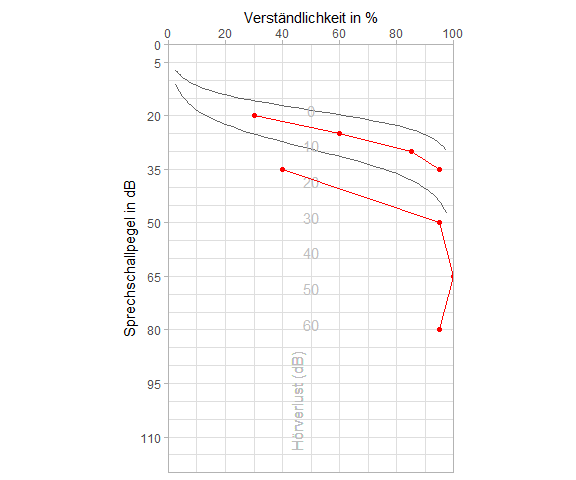# Freiburger Sprachtest

#### 11 7 2020

The Freiburger Sprachtest is arguably the most important speech audiometry test in German speaking countries. Just like pure tone audiometry it comes with an established look that can be resembled using the audiometry package in R.

Assuming some sample data

expl <- data.frame(test = gl(2,4, labels = c("Zahlen", "Einsilber")),
spl = c(20, 25, 30, 35, 35, 50, 65, 80),
int = c(30, 60, 85, 95, 40, 95, 100, 95))

a speech audiogram could be drawn like this:

library(ggplot2)
library(audiometry)
gg_freiburg(expl) +
geom_point(aes(x=spl, y=int, group=test), color="red") +
geom_line(aes(x=spl, y=int, group=test), color="red")